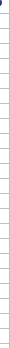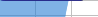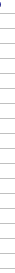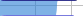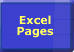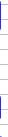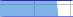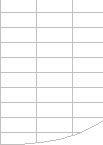# Faster Excel Calculations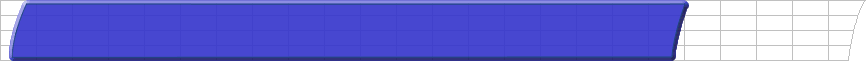Importance of Speed Faster Calculations Microsoft's Advice Bottlenecks/Size Lookups Dynamic Ranges Totalling Generating Workbooks Multi-Level Calculations Array Formulae SUMIF Example Worksheet Links## Faster Excel Calculations

Microsoft Excel can calculate millions of formulae per second.

And each new version of Excel calculates most spreadsheets faster than the previous one.

Calculation bottlenecks are the major reason for slow spreadsheets.

#### Usually its possible to speed up the calculation of slow spreadsheets, often significantly:

• Most spreadsheets contain a small number of problem areas, or Calculation Bottlenecks.
• Because Excel is such a flexible spreadsheet system there are many different formulae that can produce the answer you want.
• Some of these formulae are much faster than others.
• In a large spreadsheet it is not always easy to find and prioritise the bottlenecks.

Measure calculation time and prioritise the bottlenecks.

• Time the calculation and find the calculation bottlenecks.
• Try a different formula/method that gets the same results.
• Time the calculation again.

• Find and highlight bottlenecks.
• Optimise the worksheet calculation sequence.
• Time calculation of workbooks, worksheets and formula blocks.
• Get on-line help for calculation bottlenecks tips and techniques.
• Document calculation timings and memory usage in a consistent way.

This site provides Tips and Techniques you can apply to common bottlenecks, and tells you many little-known secrets about Excel’s calculation methods and options.

These tips, techniques and secrets have been developed by Decision Models from experience and tests over a number of years.

#### Prioritise the Calculation Bottlenecks

Use FastExcel to determine where the calculation bottlenecks are and how much calculation time each one is using. Then start with the largest!

Its not the number of formulae that consumes the calculation time, it’s the number of cell references and calculation operations.

#### Count the number of cells references and calculations required.

Work out roughly how many cell references and calculations are required for Excel to calculate the result for this bottleneck. Then think how you might get the same result with fewer references and calculations.

Move repeated calculations out to a 'helper' cell.Avoid complex mega-formulae: keep it simple and use more formulae: its generally faster to calculate.

#### Look for repeated/duplicated expressions.

Look at the bottleneck and see if you can spot expressions or calculations or references to ranges of cells that are repeated or duplicated, either within the bottleneck formulae or across a number of bottleneck formulae. Then work out how to eliminate the duplication by moving that part of the formulae to a separate 'helper' cell or cells, and replace it in the bottleneck formulae with a reference to the 'helper' cell.

#### Volatile Functions.

Volatile functions can slow down recalculation because they increase the number of formulae that must be recalculated at each calculation.
You can often reduce the number of volatile functions by using INDEX rather than OFFSET, and CHOOSE rather than INDIRECT.
But OFFSET itself is a fast function and can often be used in creative ways that give very fast calculation.

Measure and test each change.

Because each Excel Spreadsheet is unique you should:

• Measure the change in calculation time for each change you make.
• Test to make sure you are still getting the correct results after a change.© 2001-2020 Decision Models Legal Disclaimer Privacy Statement